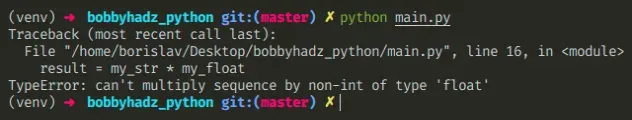# TypeError: can't multiply sequence by non-int of type floatLast updated: Apr 20, 2022

## TypeError: can't multiply sequence by non-int of type float#

The Python "TypeError: can't multiply sequence by non-int of type 'float'" occurs when we try to multiply a sequence (e.g. a string or a list) by a float. To solve the error, convert the string to a number before multiplying it, e.g. `float(my_str) * 3.14`.Here is an example of how the error occurs.

main.py
```Copied!```my_str = '2.5'
my_float = 3.1

# ⛔️ TypeError: can't multiply sequence by non-int of type 'float'
result = my_str * my_float
``````

If you have a numeric string, you have to convert it to a `float` or an `int` before multiplying it by a floating-point number.

main.py
```Copied!```my_str = '2.5'
my_float = 3.1

result = float(my_str) * my_float

print(result)  # 👉️ 7.75
``````
IMPORTANT: if you use the `input()` built-in function, all of the values the user enters get converted to strings (even numeric values).

Note that you can multiply a string and an integer, but you cannot multiply a string and a float.

main.py
```Copied!```my_str = 'ab'

my_float = 3.14

print(my_str * int(my_float))  # 👉️ "ababab"
``````

Notice that we used the `int()` class to convert the floating-point number to an integer before multiplying.

If you need to multiply each item in a list or a tuple by a number, use a list comprehension.

main.py
```Copied!```my_list = [1.1, 2.2, 3.3]

my_float = 2.2

result = [x * my_float for x in my_list]

# 👇️ [2.4200000000000004, 4.840000000000001, 7.26]
print(result)
``````

We used a list comprehension to multiply each item in the list by `2.2`.

The error often occurs when getting user input using the built-in `input()` function.

main.py
```Copied!```num = input('Enter your fav number: ')
print(num)  # 👉️ '3.14'
print(type(num))  # 👉️ <class 'str'>

# ⛔️ TypeError: can't multiply sequence by non-int of type 'float'
result = num * 2.2
``````

The input function converts the data to a string and returns it.

Even if the user enters a number, it gets converted to a string before it is returned from `input()`.

You can use the `float()` or `int()` classes to convert the string to a number.

main.py
```Copied!```num = input('Enter your fav number: ')
print(num)  # 👉️ '3.14'

result = float(num) * 2.2
print(result)  # 👉️ 6.908000000000001
``````

We converted the string to a floating-point number before multiplying by `2.2`.

Note that multiplying a sequence like a list, tuple or string by an integer is valid.

main.py
```Copied!```my_list = ['a', 'b']

my_float = 3.14

result = my_list * int(my_float)

print(result) # 👉️ ['a', 'b', 'a', 'b', 'a', 'b']
``````

However, you cannot multiply a sequence by a float.

If you aren't sure what type of object a variable stores, use the `type()` class.

main.py
```Copied!```my_list = ['a', 'b']

print(type(my_list))  # 👉️ <class 'list'>
print(isinstance(my_list, list))  # 👉️ True

my_float = 3.14

print(type(my_float))  # 👉️ <class 'float'>
print(isinstance(my_float, float))  # 👉️ True
``````

The type class returns the type of an object.

The isinstance function returns `True` if the passed in object is an instance or a subclass of the passed in class.

I wrote a book in which I share everything I know about how to become a better, more efficient programmer.You can use the search field on my Home Page to filter through all of my articles.